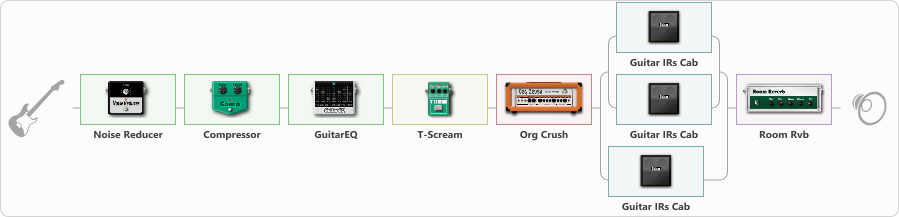# Kridvel metall 1.2v

Discussion in 'ToneLib-GFX presets' started by Kridvel, Sep 19, 2021.

1. Kridvel metall 1.2v

Preset name: Kridvel

Effects chain:Effect: "Noise Reducer" (Dynamics / Filter), active - "yes"
{
"Sens" = 85
"Mode" = Hard
}

Effect: "Compressor" (Dynamics / Filter), active - "yes"
{
"Sense" = 38
"Level" = 38
}

Effect: "GuitarEQ" (Dynamics / Filter), active - "yes"
{
"160 Hz" = 1
"400 Hz" = 2
"800 Hz" = 0
"1.6 kHz" = 2
"3.2 kHz" = 0
"6.4 kHz" = -3
"12 kHz" = 0
"Level (dB)" = 0
}

Effect: "T-Scream" (Overdrive / Distortion), active - "yes"
{
"Drive" = 48
"Tone" = 80
"Level" = 100
}

Effect: "Org Crush" (Amp simulators), active - "yes"
{
"Gain" = 61
"Bass" = 54
"Middle" = 56
"Treble" = 56
"Presence" = 59
"Master" = 53
"Level (dB)" = 3
}

Effect: "Splitter" (Dynamics / Filter)
{
"A-Bypass" = Off
"A-Pan" = 0
"A-Level" = 55
"B-Bypass" = Off
"B-Pan" = 0
"B-Level" = 55

'A' branch:
{

Effect: "Splitter" (Dynamics / Filter)
{
"A-Bypass" = Off
"A-Pan" = 0
"A-Level" = 55
"B-Bypass" = Off
"B-Pan" = 0
"B-Level" = 55

'A' branch:
{

Effect: "Guitar IRs Cab" (Cabinets), active - "yes"
{
"Model" = Marshall 1960A (4x12")
"Mic Position" = Center
"Mic Distance" = Near
"Low Cut (Hz)" = 80
"Hi Cut (kHz)" = 9.0
"Mix" = 100
"Level (dB)" = 2
}
}
'B' branch:
{

Effect: "Guitar IRs Cab" (Cabinets), active - "yes"
{
"Model" = Marshall 1960b (4x12")
"Mic Position" = Middle
"Mic Distance" = Far
"Low Cut (Hz)" = 88
"Hi Cut (kHz)" = 10.0
"Mix" = 100
"Level (dB)" = 2
}
}
}
}
'B' branch:
{

Effect: "Guitar IRs Cab" (Cabinets), active - "yes"
{
"Model" = Orange PPC (4x12")
"Mic Position" = Edge
"Mic Distance" = Middle
"Low Cut (Hz)" = 73
"Hi Cut (kHz)" = 9.0
"Mix" = 100
"Level (dB)" = 4
}
}
}

Effect: "Room Rvb" (Reverberation), active - "yes"
{
"Time" = 3.4
"PreDelay" = 0
"LoDamp" = 16
"HiDamp" = 13
"Mix" = 57
}

Note: You will need to download and install the ToneLib-GFX software to use the preset.

File size:
1 KB
Views:
2,501